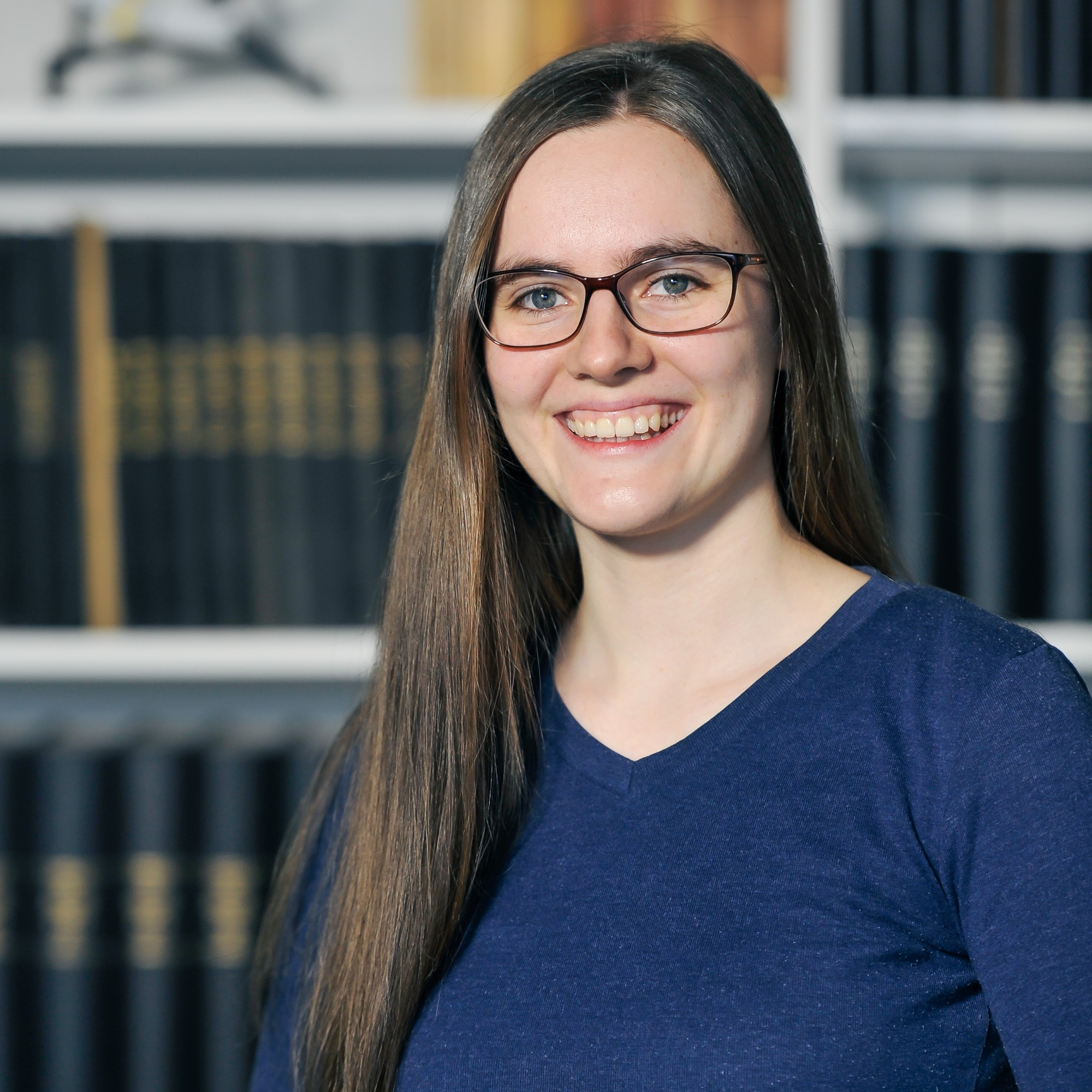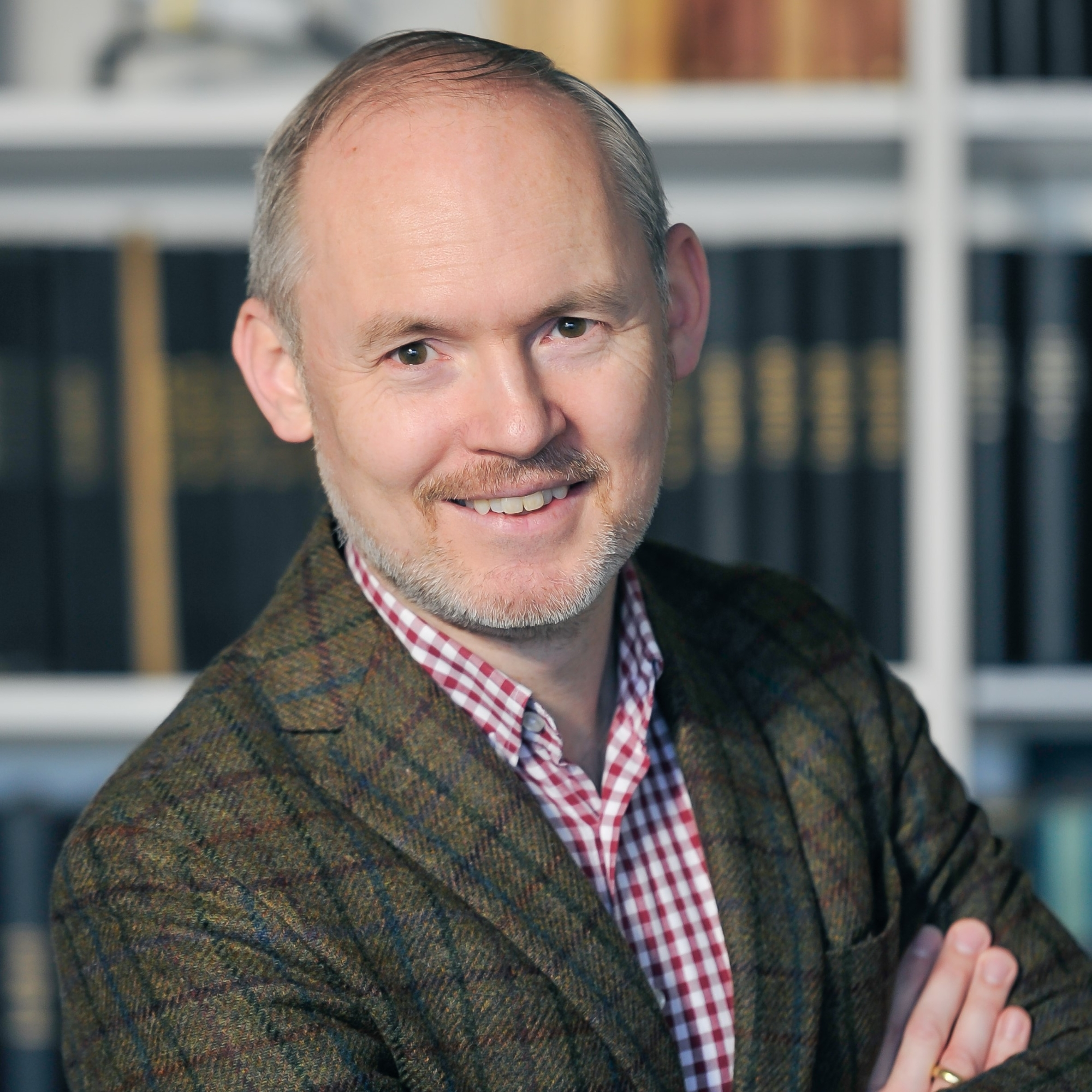# Koopman-based stability analysis

The aim of this project is to utilize the Koopman framework to arrive at accurate and computationally efficient stability methods for classes of linear and nonlinear dynamical systems.

The Koopman operator provides a way to approximate the dynamics of a nonlinear system by a linear time-invariant system of higher order. If the Koopman framework is applied to time-autonomous systems, the approximate linear dynamics obtained by the Koopman lift then takes a linear autonomous form.

The incorporation of a time-dependent input into the dynamics generally poses problems in the Koopman framework as the system can only be approximated by a linear time-invariant system if products of state and input are neglected.

In this project we introduce a specific choice of Koopman basis functions combining the Taylor and Fourier bases, which allows to recover all equations necessary to perform the Harmonic Balance Method as well as the Hill analysis directly from the linear lifted dynamics. This lifted dynamics can then be used to interpret the Hill matrix as a system matrix for a linear system, opening a different route to stability information, which shares some properties both with classical frequency-based and with classical time-based stability techniques.

### Journal articles

1. Bayer, F., & Leine, R. I. (2023). Sorting-free Hill-based stability analysis of periodic solutions through Koopman analysis. Nonlinear Dynamics, 111, 8439–8466. https://doi.org/10.1007/s11071-023-08247-7

### Proceedings

1. Bayer, F., & Leine, R. I. (2023, Juni). Optimal projection in a Koopman-based sorting-free Hill method. Proceedings of the Third International Nonlinear Dynamics Conference (NODYCON 2023). https://nodycon.org/2023/
2. Bayer, F., & Leine, R. I. (2022, Juli). A Koopman view on the harmonic balance and Hill method. Proceedings of the 10th European Nonlinear Dynamics Conference (ENOC2020+2). https://enoc2020.sciencesconf.org/394116/

### Kontakt### Fabia Bayer

M.Sc.### Remco I. Leine

Prof. Dr. ir. habil.

Direktor